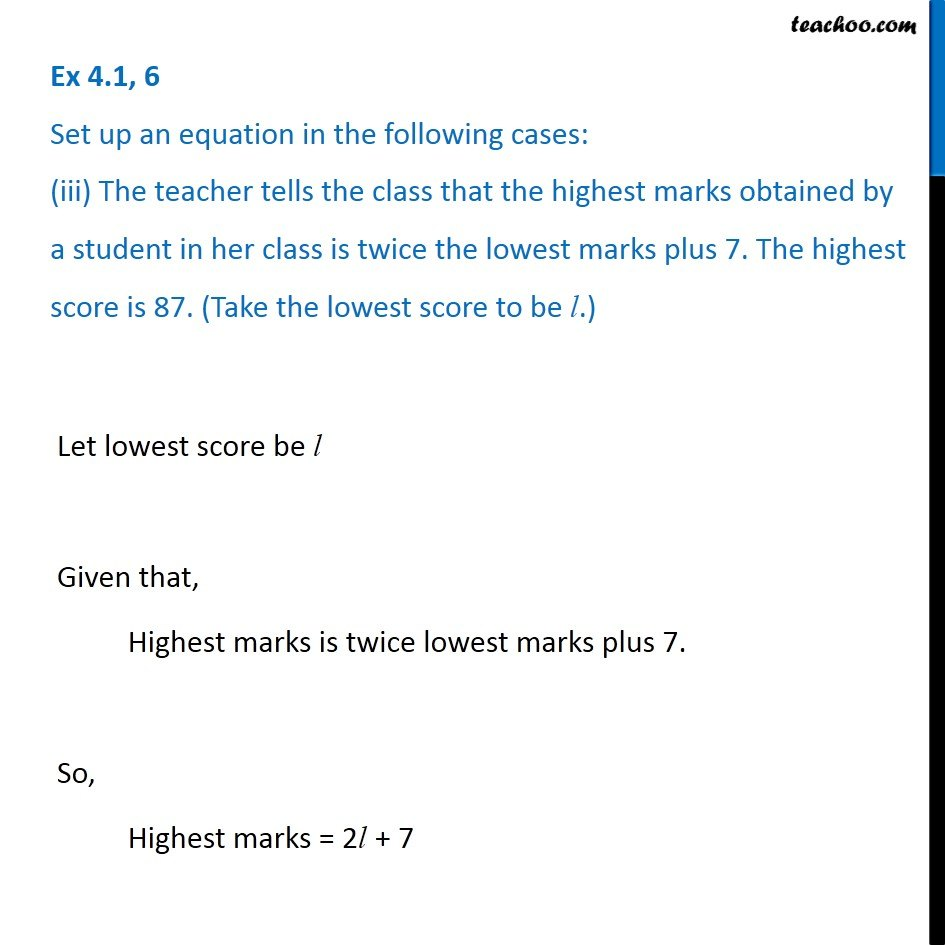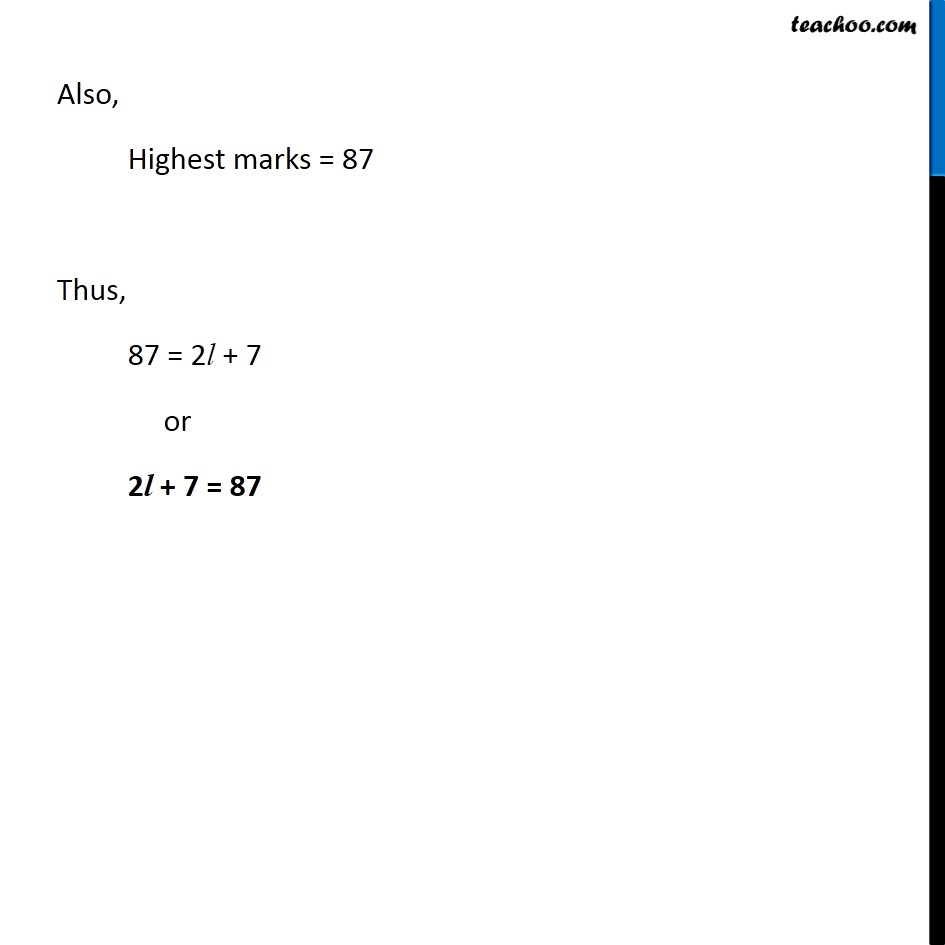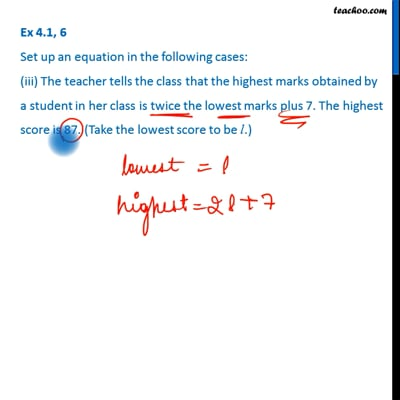Ex 4.1

Chapter 4 Class 7 Simple Equations
Serial order wiseThis video is only available for Teachoo black users

Learn in your speed, with individual attention - Teachoo Maths 1-on-1 Class

### Transcript

Ex 4.1, 6 Set up an equation in the following cases: (iii) The teacher tells the class that the highest marks obtained by a student in her class is twice the lowest marks plus 7. The highest score is 87. (Take the lowest score to be l.) Let lowest score be l Given that, Highest marks is twice lowest marks plus 7. So, Highest marks = 2l + 7 Also, Highest marks = 87 Thus, 87 = 2l + 7 or 2l + 7 = 87